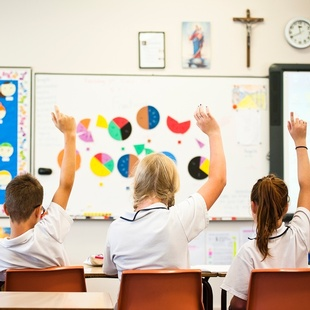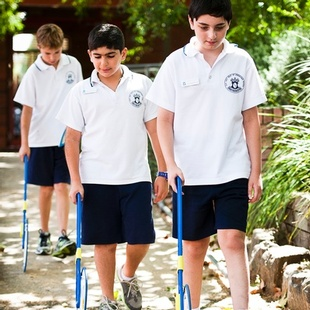Mathematics - Our Lady Help of Christians, Epping

# Mathematics

The aim of Mathematics at Our lady Help of Christians is to develop students’ mathematical thinking, understanding and confidence in the application of Mathematics.Mathematics involves the development of students’ thinking, understanding, competence and confidence in the application of mathematics. The five broad strands of Mathematics are:

• Working Mathematically
• Number
• Patterns and Algebra
• Measurement and Data
• Space and Geometry